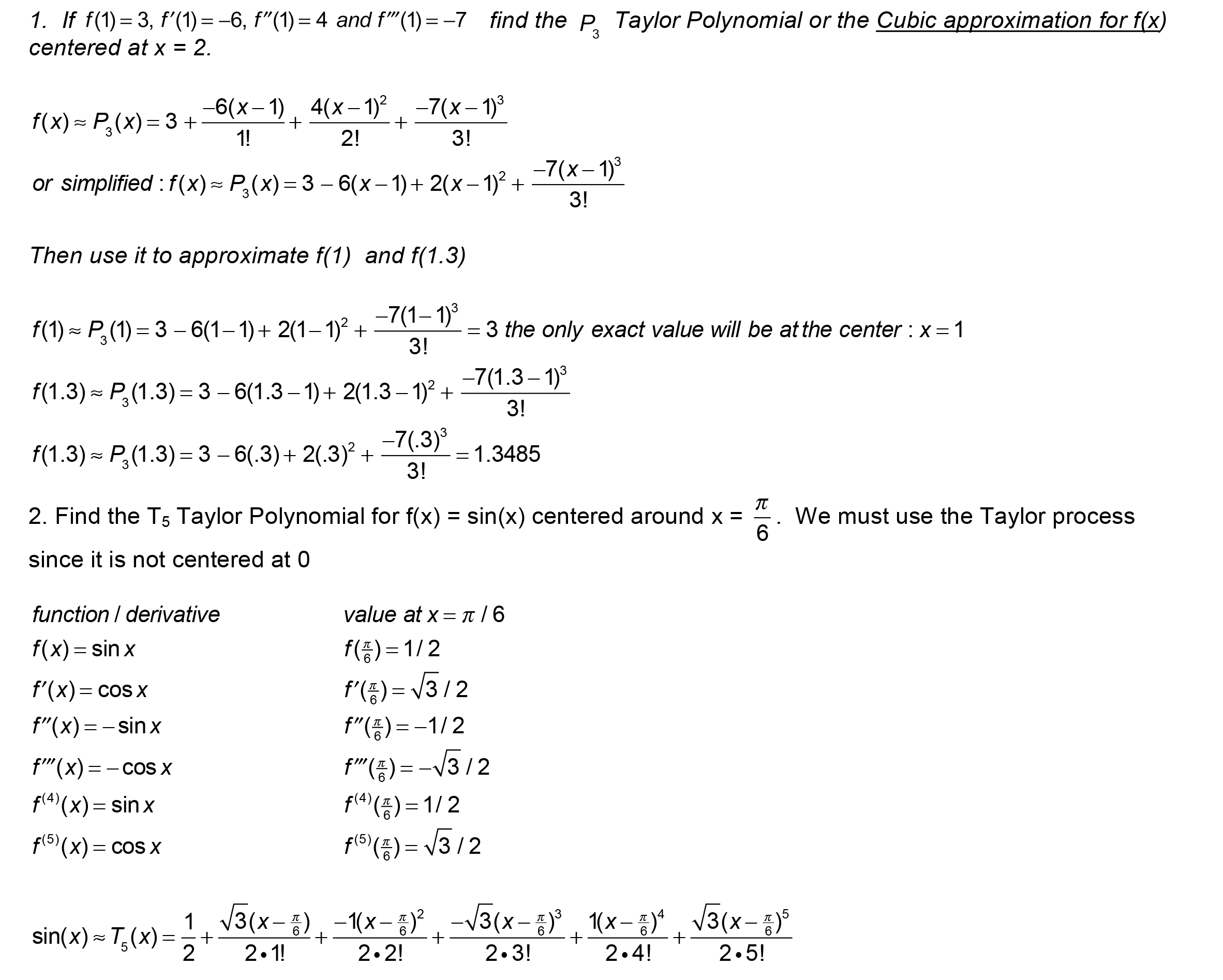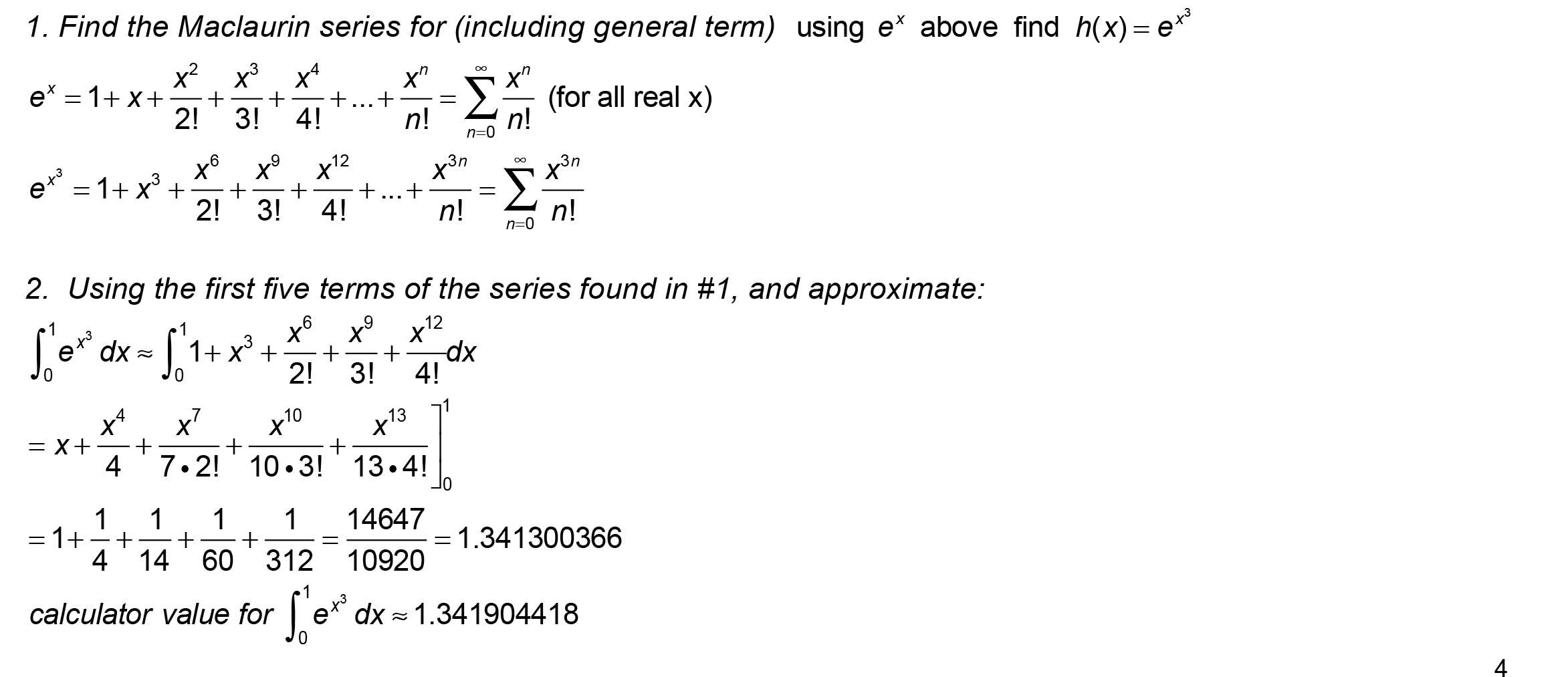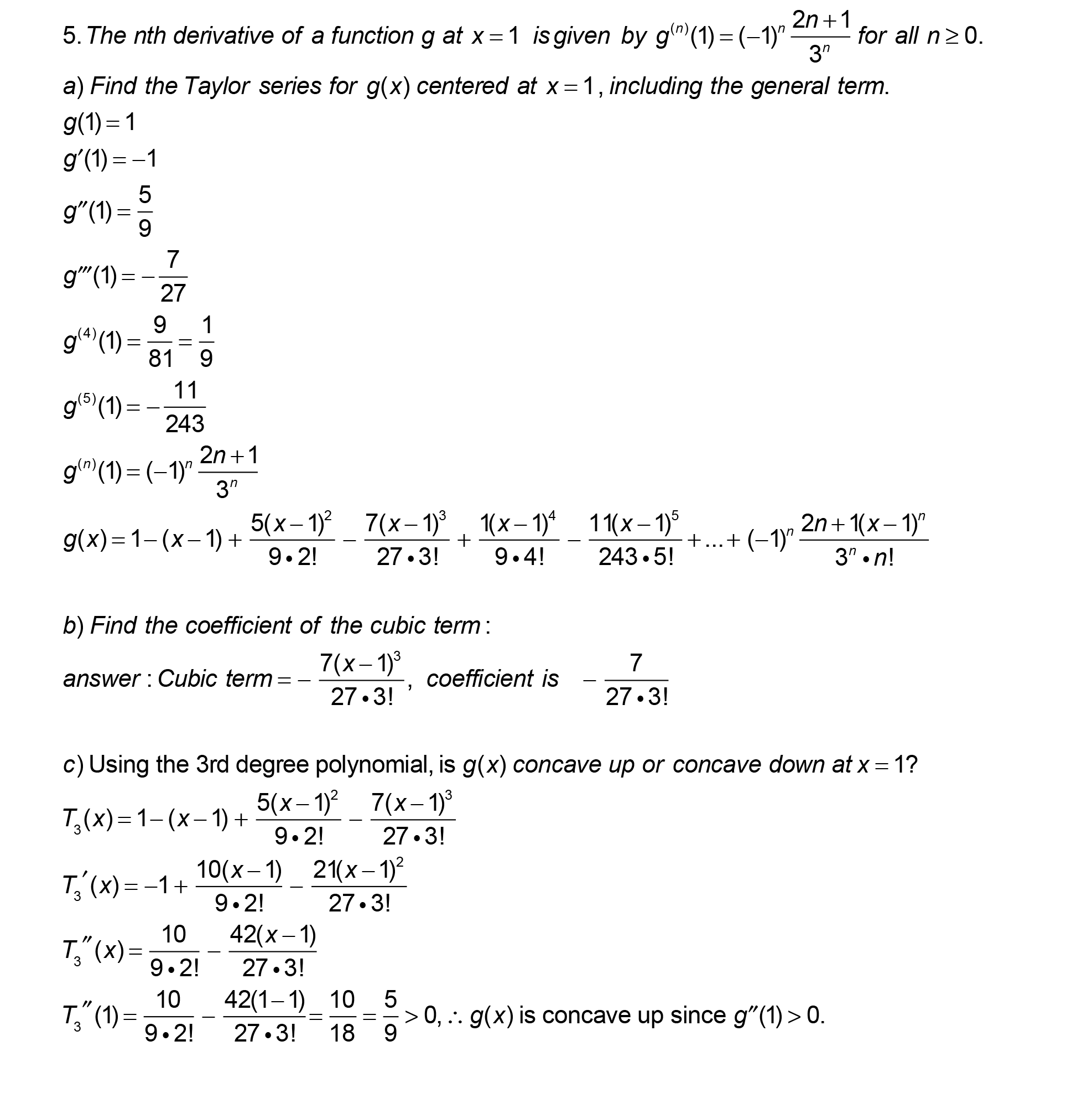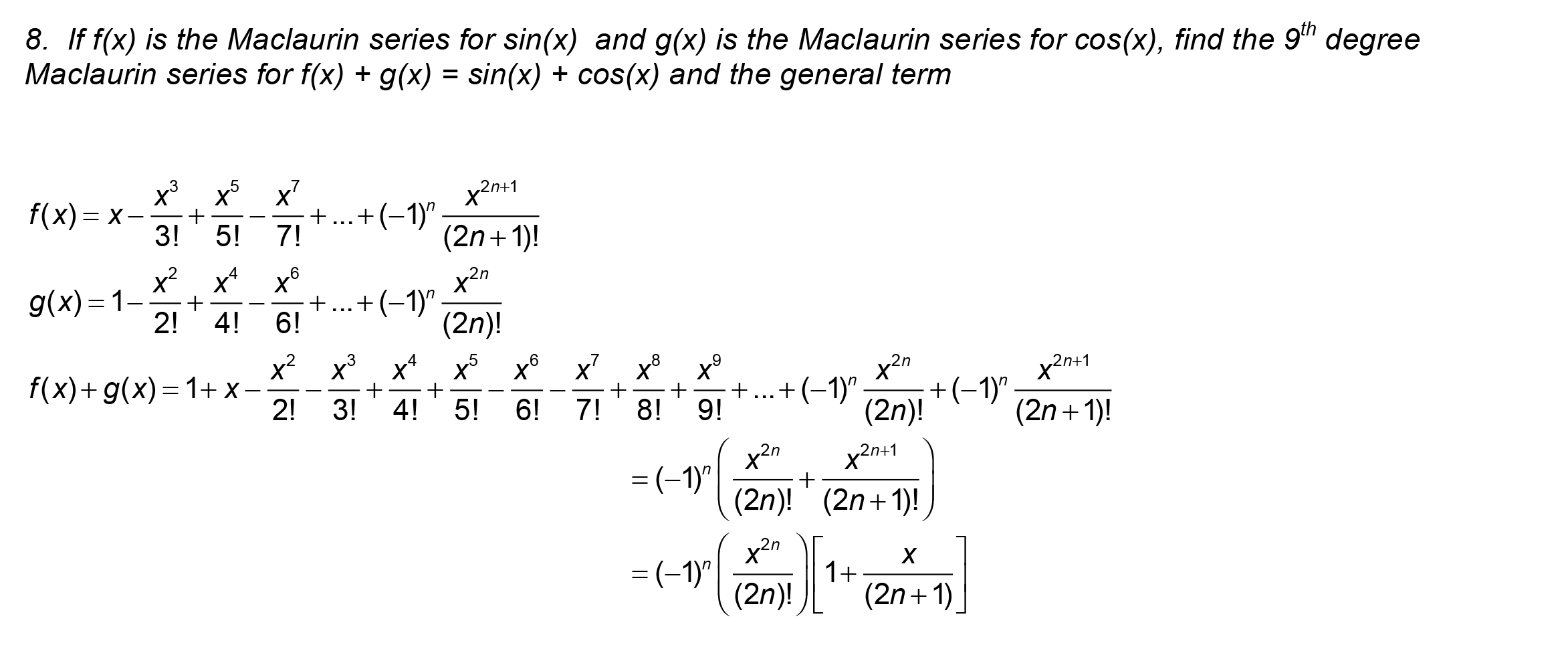10.2 Taylor SeriesFind Taylor Polynomial.Find Maclaurin for cos(x)Find Maclaurin for e^(x)Find Maclaurin for e^(x^3) and integrate it.Find Maclaurin for 3x•sin(x^2)Find Maclaurin for cos(x^(3/2)) and use it to solve a differential equation.Find a Taylor given a derivative generatorCombining two Maclaurins